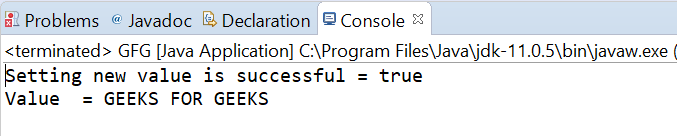Open in App
Not now

# AtomicReference weakCompareAndSetVolatile() method in Java with Examples

• Last Updated : 27 Dec, 2019

The weakCompareAndSetVolatile() method of a AtomicReference class is used to atomically sets the value to newValue for AtomicReference if the current value is equal to expectedValue passed as parameter. This method updates the value with memory effects as specified by VarHandle.weakCompareAndSet(java.lang.Object…). This method returns true if set a new value to AtomicRefrence is successful.

Syntax:

```public final boolean
weakCompareAndSetVolatile(V expectedValue,
V newValue)
```

Parameters: This method accepts expectedValue which is the expected value and newValue which is the new value to set.

Return value: This method returns true if successful.

Below programs illustrate the weakCompareAndSetVolatile() method:
Program 1:

 `// Java program to demonstrate ` `// AtomicReference.weakCompareAndSetVolatile() method ` ` `  `import` `java.util.concurrent.atomic.AtomicReference; ` ` `  `public` `class` `GFG { ` `    ``public` `static` `void` `main(String[] args) ` `    ``{ ` ` `  `        ``// create an atomic reference object. ` `        ``AtomicReference ref ` `            ``= ``new` `AtomicReference(); ` ` `  `        ``// set some value ` `        ``ref.set(``1234.00``); ` ` `  `        ``// apply weakCompareAndSetVolatile() ` `        ``boolean` `result ` `            ``= ref.weakCompareAndSetVolatile(``124.00``, ` `                                            ``234.32``); ` ` `  `        ``// print value ` `        ``System.out.println(``"Setting new value"` `                           ``+ ``" is successful = "` `                           ``+ result); ` ` `  `        ``System.out.println(``"Value = "` `+ ref.get()); ` `    ``} ` `} `

Output:Program 2:

 `// Java program to demonstrate ` `// AtomicReference.weakCompareAndSetVolatile() method ` ` `  `import` `java.util.concurrent.atomic.AtomicReference; ` ` `  `public` `class` `GFG { ` `    ``public` `static` `void` `main(String[] args) ` `    ``{ ` ` `  `        ``// create an atomic reference object which stores String. ` `        ``AtomicReference ref = ``new` `AtomicReference(); ` ` `  `        ``// set some value ` `        ``ref.set(``"GFG"``); ` ` `  `        ``// apply weakCompareAndSetVolatile() ` `        ``boolean` `result ` `            ``= ref.weakCompareAndSetVolatile( ` `                ``"GFG"``, ` `                ``"GEEKS FOR GEEKS"``); ` ` `  `        ``// print value ` `        ``System.out.println(``"Setting new value"` `                           ``+ ``" is successful = "` `                           ``+ result); ` `        ``System.out.println(``"Value  = "` `+ ref.get()); ` `    ``} ` `} `

Output:My Personal Notes arrow_drop_up
Related Articles